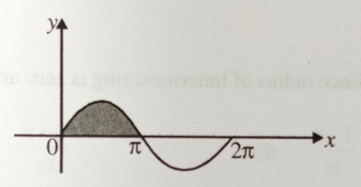$\mathrm{A}=4\mathrm{i}+4\mathrm{j}-4\mathrm{k}$ and $\mathrm{B}=3\mathrm{i}+\mathrm{j}+4\mathrm{k}$, then angle between vectors A and B is:

(A)  $180°$

(B)  $90°$

(C)  $45°$

(D)  $0°$

Concept Questions :-

Resultant of Vectors
High Yielding Test Series + Question Bank - NEET 2020

Difficulty Level:

If the magnitude of sum of two vectors is equal to the magnitude of difference of the two vectors, the angle between these vectors is

Concept Questions :-

Resultant of Vectors

Difficulty Level:

If vectors $A=\mathrm{cos}\omega \stackrel{^}{i}+\mathrm{sin}\omega \stackrel{^}{j}$ and are functions of time, then the value of t at which they are orthogonal to each other

1. $t=\frac{\mathrm{\pi }}{4\omega }$

2. $t=\frac{\mathrm{\pi }}{2\omega }$

3. $t=\frac{\mathrm{\pi }}{\omega }$

4. $t=0$

Concept Questions :-

Scalar Product

Difficulty Level:

The angle between two vectorsandis

1.  ${\mathrm{cos}}^{-1}\left(\frac{1}{3}\right)$

2.  ${\mathrm{cos}}^{-1}\left(\frac{-1}{3}\right)$

3.  ${\mathrm{sin}}^{-1}\left(\frac{-1}{3}\right)$

4.  $90°$

Concept Questions :-

Scalar Product

Difficulty Level:

The distance x (in) covered by a molecule starting from point A at time t = 0 and stopping at another point B in given by the equationThe distance between A and B (in) is closed to

1.  10.7

2.  20.7

3.  40.7

4.  50.7

Concept Questions :-

Differentiation

Difficulty Level:

A curve is governed by the equation y=sinx, then what is the area enclosed by the curve and x-axis between x =0 and x =$\mathrm{\pi }$ is (shaded region)1. 1 units

2. 2 units

3. 3 units

4. 4 units

Concept Questions :-

Integration
High Yielding Test Series + Question Bank - NEET 2020

Difficulty Level:

Calculate the area of disk of radius 'a' using integration

1. $\frac{{\mathrm{\pi a}}^{2}}{2}$

2. ${\mathrm{\pi a}}^{2}$

3. $\frac{3}{2{\mathrm{\pi a}}^{2}}$

4. $2{\mathrm{\pi a}}^{2}$

Concept Questions :-

Integration
High Yielding Test Series + Question Bank - NEET 2020

Difficulty Level:

The acceleration of a particle starting from rest varies with time according to relation, . Find the velocity of the particle at time instant t.

1. $\alpha {t}^{2}+\beta t$

2. $\alpha {t}^{2}+\frac{\beta t}{2}$

3. $\frac{\alpha {t}^{2}}{2}+\beta t$

4. $2\alpha {t}^{2}+\beta t$

Concept Questions :-

Integration
High Yielding Test Series + Question Bank - NEET 2020

Difficulty Level:

The displacement of particle is zero at t=0 and at t=t it is x. It starts moving in the x direction with velocity, which varies as $v=k\sqrt{x}$, where k is constant. The velocity-

1. varies with time

2. is independent to time

3. inversely proportional to time

4. inversely proportional to acceleration

Concept Questions :-

Integration
High Yielding Test Series + Question Bank - NEET 2020

Difficulty Level:

The acceleration of a particle is given as $a=3{x}^{2}$. At t=0, v=0, x=0, the velocity at t =2 sec will be-

1.  0.05 m/s

2. 0.5 m/s

3. 5 m/s

4. 50 m/s

Concept Questions :-

Integration